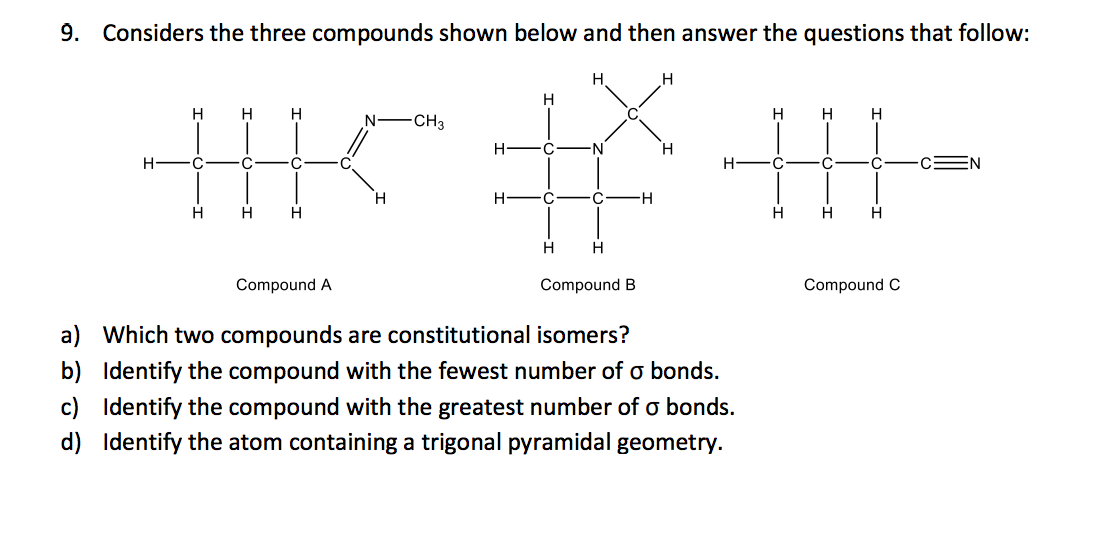# 9. Considers the three compounds shown below and then answer the questions that follow: H H...

###### Question:9. Considers the three compounds shown below and then answer the questions that follow: H H H H H H H N-CH3 H H C N H H: C C H: [ C CSN H HC C H H H H H H H H H Compound A Compound B Compound C a) Which two compounds are constitutional isomers? b) Identify the compound with the fewest number of o bonds. c) Identify the compound with the greatest number of o bonds. d) Identify the atom containing a trigonal pyramidal geometry.

#### Similar Solved Questions

At Dot Com, a large retailer of popular books, demand is constant at 20,400 books per year. The cost of placing an order to replenish stock is $75, and the annual cost of holding is$5.00 per book. Stock is received 15 working days after an order has been placed. No backordering is allowed. Assume 2...
##### What is the derivative of y=1/log_2(x)?
What is the derivative of y=1/log_2(x)?...